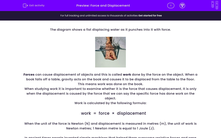# Define and Calculate Work Done

In this worksheet, students will learn about work done by a force and how to calculate it using the displacement it causes.Key stage:  KS 3

Curriculum topic:   Physics: Energy

Curriculum subtopic:   Energy Changes and Transfers

Difficulty level:#### Worksheet Overview

The diagram shows a fist displacing water as it punches into it with force.Forces can cause displacement of objects and this is called work done by the force on the object. When a book falls off a table, gravity acts on the book and causes it to be displaced from the table to the floor. This means work was done on the book. When a force moves an object, energy is transferred and this means that work has been done.

When studying work it is important to examine if it is the force that causes displacement. It is only when the displacement is caused by the force that we can say the specific force has done work on the object.

Work is calculated by the following formula:

work  =  force  ×  displacement

When the unit of the force is Newton (N) and displacement is measured in metres (m), the unit of work is Newton metres.

1 Newton metre is equal to 1 joule (J).

In ancient times, people invented simple machines that helped them to overcome resistive forces so that they were able to do work that seemed impossible, like building the pyramids in Egypt.Simple machines work in the following ways:

Transfer a force from one place to another

Change the direction of a force

Increase the magnitude of a force

Increase the displacement the force causes

Change the speed of the force

Examples of simple machines are the lever, the wheel and axle, the pulley, the inclined plane, the wedge and the screw.

A machine produces a mechanical advantage when the force applied by the person using it (input force) is smaller than the force applied to the task (output force).### What is EdPlace?

We're your National Curriculum aligned online education content provider helping each child succeed in English, maths and science from year 1 to GCSE. With an EdPlace account you’ll be able to track and measure progress, helping each child achieve their best. We build confidence and attainment by personalising each child’s learning at a level that suits them.

Get started